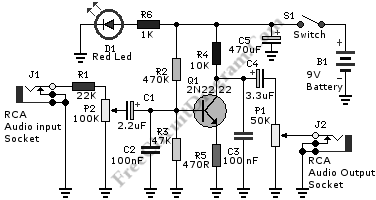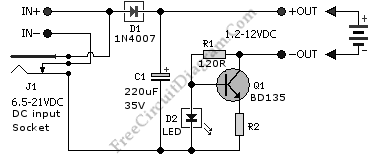# Low Cost Universal Battery ChargerThis is a Low Cost Universal Battery Charger Circuit. This circuit is used to charge NiCD-NiMH batteries and ideal for car use. This circuit transforms a mains adapter to a charger. It can be used to charge MP3 players, portables, toys cellular phone, video batteries. This circuit also has selectable charge current. To indicate this device is charging, an LED is used in this circuit. Here is the circuit:The charge current of this circuit is depends on the value of R2. If the charge current is 50mA, R2 that is used is 47R. Using R2, 18R, the charge current of this circuit is 100mA. If the charge current is 200mA, R2 that is used is 6.8R. with R2 3.9R, the charge current will be 300mA and if the charge current is 400mA, R2 should be 2.7R. This charger should be used with caution: the battery might be overcharged, turn off the charging after the battery voltage indicate the fully charged level. You better connect a voltmeter in parallel with the battery and watchout! [Circuit’s schematic diagram source: extremecircuits.net]